﻿ 佘山地震基准台四分量钻孔应变仪相对标定文章快速检索
 地震地磁观测与研究2020, Vol. 41Issue (3): 118-125  DOI: 10.3969/j.issn.1003-3246.2020.03.0160

### 引用本文NI Youzhong, YE Qing, ZHONG Weixing, et al. Field relative calibration of four-component borehole strain gauge at Sheshan Seismic Station[J]. Seismological and Geomagnetic Observation and Research, 2020, 41(3): 118-125. DOI: 10.3969/j.issn.1003-3246.2020.03.016.### 文章历史

1. 中国上海 201602 佘山地震基准台;
2. 中国上海 201602 上海佘山地球物理国家野外科学观测研究站

Field relative calibration of four-component borehole strain gauge at Sheshan Seismic Station
NI Youzhong, YE Qing , ZHONG Weixing , ZHU Guohao , FANG Tao , JIAO Congcong , WU Shanshan
1. Sheshan Seismic Reference Station, Shanghai 201602, China;
2. Shanghai Sheshan National Geophysical Observatory and Research Station, Shanghai 201602, China
Abstract: The observation data of the YRY-4 four-component borehole strain gauge basically conforms to a self-consistent equation. When observation data does not conform to the self-consistent equation, one possible reason is that the sensitivity of the components in the downhole probe is changed. The four-component borehole strain gauge at Sheshan Seismic Station was installed in 2006 and has been operating for more than 10 years. The components in the downhole probe aged to different degrees. Therefore, a field relative calibration must be carried out before data analysis. In this paper, the sensitivity coefficient K of the four components and the reliability index of C95 before and after correction are calculated by using original values and difference values of the observation data at a monthly scale and an annual scale. It is found that at the monthly scale, if the observation data is in good quality, the effect of correction on original values is better; when there are some "bad points", the correction on difference values can effectively remove the bad points and improve the reliability index of C95. At the annual scale, the C95 reliability index is higher after correction on original values.
Key words: borehole strain observation    C95 reliability    field relative calibration    self-consistent
0 引言

YRY-4型四分量钻孔应变仪观测数据基本符合自洽方程，当数据不符合自洽方程时，一个可能原因是，井下探头中元件的灵敏度发生了变化。邱泽华等（2005）易志刚等（2007）阚宝祥等（2007）吴立辛等（2010）柳忠旺等（2014）均在研究中提及，可利用四分量钻孔应变仪互相垂直方向应力之和为不变量的特点，依次以每个元件为标准，分别计算各元件灵敏度。

1 基本公式

 ${S_\theta } = A({\varepsilon _1} + {\varepsilon _2}) + B({\varepsilon _1} - {\varepsilon _2}){\rm{cos}}{\kern 1pt} {\kern 1pt} 2(\theta - \varphi )$ (1)

 ${S_i} = {K_i}{\kern 1pt} {R_i}$ (2)

 $\left\{ \begin{array}{*{35}{l}} {{S}_{1}}={{S}_{{{\theta }_{1}}}}=A({{\varepsilon }_{1}}+{{\varepsilon }_{2}})+B({{\varepsilon }_{1}}-{{\varepsilon }_{2}})\text{cos}2({{\theta }_{1}}-\varphi ) \\ {{S}_{2}}={{S}_{{{\theta }_{1}}+{}^{\pi }\!\!\diagup\!\!{}_{4}\;}}=A({{\varepsilon }_{1}}+{{\varepsilon }_{2}})-B({{\varepsilon }_{1}}-{{\varepsilon }_{2}})\text{sin}2({{\theta }_{1}}-\varphi ) \\ {{S}_{3}}={{S}_{{{\theta }_{1}}+{}^{\pi }\!\!\diagup\!\!{}_{2}\;}}=A({{\varepsilon }_{1}}+{{\varepsilon }_{2}})-B({{\varepsilon }_{1}}-{{\varepsilon }_{2}})\text{cos}2({{\theta }_{1}}-\varphi ) \\ {{S}_{4}}={{S}_{{{\theta }_{1}}+{}^{3\pi }\!\!\diagup\!\!{}_{4}\;}}=A({{\varepsilon }_{1}}+{{\varepsilon }_{2}})+B({{\varepsilon }_{1}}-{{\varepsilon }_{2}})\text{sin}2({{\theta }_{1}}-\varphi ) \\ \end{array} \right.$ (3)

 ${{S_1} + {S_3} = {S_2} + {S_4}}$ (4)

 ${{K_1}{R_1} - {K_2}{R_2} + {K_3}{R_3} - {K_4}{R_4} = 0}$ (5)

 ${{K_{11}}{R_1} - {K_{21}}{R_2} + {K_{31}}{R_3} - {K_{41}}{R_4} = 0}$ (6)
 ${{K_{12}}{R_1} - {K_{22}}{R_2} + {K_{32}}{R_3} - {K_{42}}{R_4} = 0}$ (7)
 ${{K_{13}}{R_1} - {K_{23}}{R_2} + {K_{33}}{R_3} - {K_{43}}{R_4} = 0}$ (8)
 ${{K_{14}}{R_1} - {K_{24}}{R_2} + {K_{34}}{R_3} - {K_{44}}{R_4} = 0}$ (9)

 ${K_i} = \frac{1}{4}\sum\limits_{j = 1}^4 {{K_{ji}}} {\kern 1pt} {\kern 1pt} {\kern 1pt} {\kern 1pt} {\kern 1pt} {\kern 1pt} {\kern 1pt} {\kern 1pt} {\kern 1pt} {\kern 1pt} {\kern 1pt} {\kern 1pt} {\kern 1pt} {\kern 1pt} {\kern 1pt} {\kern 1pt} (i = 1,2,3,4)$ (10)

 ${C_{95}} = 1 - \frac{{m{S_c}}}{{m{S_a}}} = 1 - \frac{{\sum\limits_{{N_{95}}} | ({S_1} + {S_3}) - ({S_2} + {S_4})|}}{{\frac{1}{2}\sum\limits_{{N_{95}}} | ({S_1} + {S_3} + {S_2} + {S_4})|}}$ (11)

2 计算结果

2.1 按月进行对比计算表 1 变化值、差分值月尺度实地相对标定和数据矫正结果 Table 1 Results of field relative calibration and data correction for original values and difference values at a monthly scale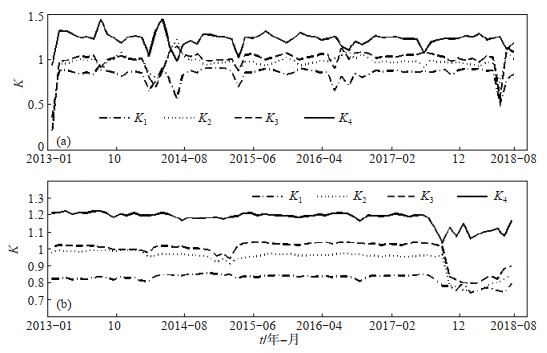图 1 变化值、差分值月尺度相对标定结果 (a)变化值实地标定；(b)差分值相对标定 Fig.1 Relative calibration results for original values and difference values at a monthly scale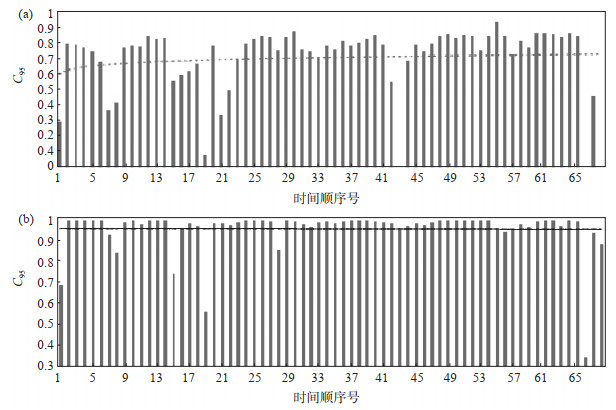图 2 各元件变化值月尺度矫正前后信度值C95 (a)矫正前；(b)矫正后 Fig.2 The reliability index of C95 for the original values of each component before and after correction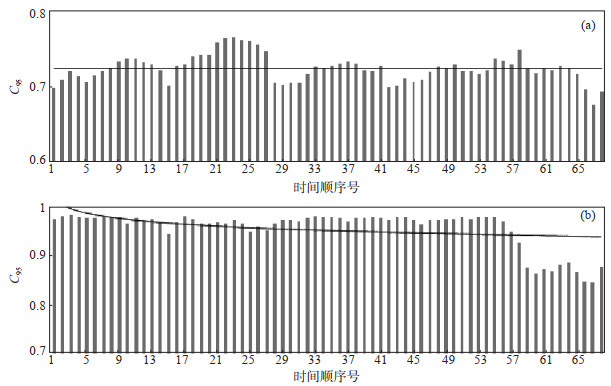图 3 各元件差分值月尺度矫正前后信度值C95 (a)矫正前；(b)矫正后 Fig.3 The reliability index of C95 for the difference values of each component before and after correction

2.2 按年进行对比计算表 2 2013—2018年变化值、差分值年尺度实地相对标定和数据矫正结果 Table 2 Results of field relative calibration and data correction for originalvalues and difference values at an annual scale from 2013 to 2018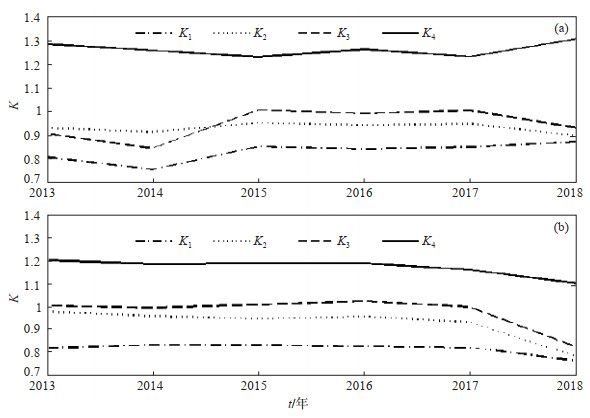图 4 2013—2018年变化值、差分值年度相对标定结果 (a)变化值；(b)差分值 Fig.4 Relative calibration results for original values and difference values at an annual scale from 2013 to 2018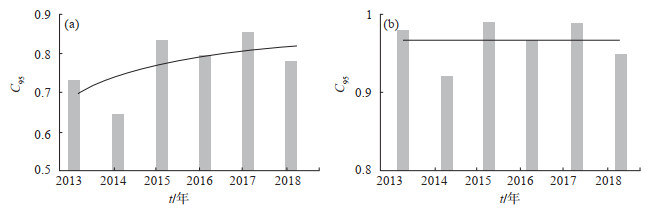图 5 变化值年度矫正前后C95 (a)矫正前；(b)矫正后 Fig.5 The C95 for original values before and after annual correction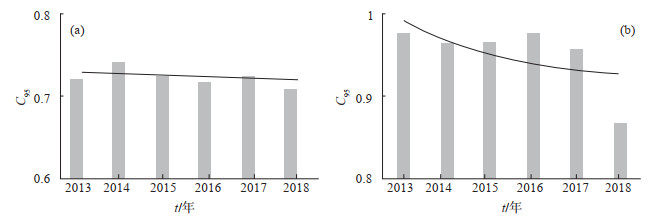图 6 差分值年度矫正前后C95 (a)矫正前；(b)矫正后 Fig.6 The C95 for difference values before and after annual correction

3 结论

（1）按月进行矫正计算时，矫正前C95＜0.6，属“坏点”较多月份，采用差分矫正，可去除突跳和台阶点，平滑曲线，有效提高C95信度指标；对于矫正前C95＞0.7的观测月，因该月观测资料质量良好，采用变化矫正方法效果更理想。

（2）按年对观测资料进行矫正时，采用变化矫正效果更优。

 阚宝祥, 邱泽华, 唐磊. 2007. 泰安台钻孔差应变观测的实地标定[J]. 大地测量与地球动力学, 27(6): 119-123. 柳忠旺, 侯炳正, 常兴, 等. 2014. 海原小山四分量钻孔应变观测相对标定和异常判定[J]. 防灾减灾学报, 30(4): 13-19. 邱泽华, 石耀霖, 欧阳祖熙. 2005. 四分量钻孔应变观测的实地相对标定[J]. 大地测量与地球动力学, 25(1): 118-122. 邱泽华. 2017. 钻孔应变观测理论和应用[M]. 北京: 地震出版社. 吴立辛, 张立强, 李国斌, 等. 2010. 海原台四分量钻孔应变观测的相对标定及资料应用[J]. 地震研究, 33(4): 318-322. DOI:10.3969/j.issn.1000-0666.2010.04.011 易志刚, 邱泽华, 宋茉. 2007. 钻孔体应变观测资料的实地校正[J]. 地震, 27(3): 117-123.